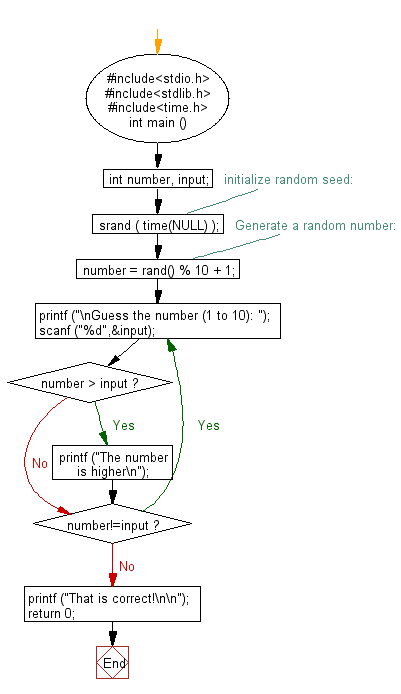﻿ C Program: Generate a random number - w3resource# C Exercises: Generate a random number

## C Variable Type : Exercise-5 with Solution

Write a C program to generate a random number.

Sample Solution:

C Code:

``````#include<stdio.h>
#include<stdlib.h>
#include<time.h>

int main ()
{
int number, input;
/* initialize random seed: */
srand ( time(NULL) );
/* Generate a random number: */
number = rand() % 10 + 1;
do {
printf ("\nGuess the number (1 to 10): ");
scanf ("%d",&input);
if (number > input)
printf ("The number is higher\n");
} while (number!=input);
printf ("That is correct!\n\n");
return 0;
}
```
```

Sample Output:

```Guess the number (1 to 10): 6
The number is higher
```

Flowchart:C Programming Code Editor:

Improve this sample solution and post your code through Disqus

What is the difficulty level of this exercise?

Test your Programming skills with w3resource's quiz.

﻿

## C Programming: Tips of the Day

Why do C and C++ compilers allow array lengths in function signatures when they're never enforced?

It is a quirk of the syntax for passing arrays to functions.

Actually it is not possible to pass an array in C. If you write syntax that looks like it should pass the array, what actually happens is that a pointer to the first element of the array is passed instead.

Since the pointer does not include any length information, the contents of your [] in the function formal parameter list are actually ignored.

Ref : https://bit.ly/3fhlvdH